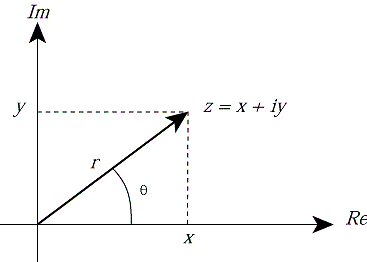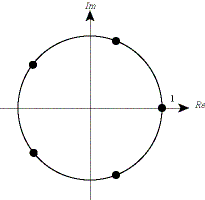EECS20N: Signals and Systems

# Polar coordinates

The representation of a complex number as a sum of a real and imaginary number, z = x + iy, is called its Cartesian representation.

Recall from trigonometry that if x, y, r are real numbers and r 2 = x 2 + y 2, then there is a unique number θ with 0 ≤  θ  <  2π such that

cos(θ) = x / r,

sin(θ) = y / r.

That number is

 θ = cos -1(x / r), = sin -1(y / r) = tan -1(y / x)

We can therefore express any complex number z = x + iy as

 z = | z | (x / | z | + iy / | z |) = | z | (cos θ + i sin θ) = | z | e iθ ,

where θ = tan -1(y / x). The angle or argument θ is measured in radians, and it is written as arg(z). So we have the polar representation of any complex number z as

z = x + iy = re iθ.

The two representations are related by

r = | z | = √(x 2 + y 2).

and

θ = arg(z) = tan -1(y / x).

The values x and y are called the Cartesian coordinates of z, while r and θ are its polar coordinates. Note that r is real and r 3 0.Note that for any integer K,

re i(2Kπ + θ) = re iθ.

This is because

re i(2Kπ + θ) = re i2Kπ e iθ

and

e i2Kπ = cos (2Kπ) + i sin (2Kπ) = 1.

Thus, the polar coordinates (r, θ) and (r, θ + 2Kπ) for any integer K represent the same complex number. Thus, the polar representation is not unique; by convention, a unique polar representation can be obtained by requiring that the angle given by a value of θ satisfying 0 ≤ θ < 2π or -π < θ ≤ π.

### Example 1

 The polar representation of the number 1 is 1 = 1 e i0. Notice that it is also true that 1 = 1 e i2π, because the sine and cosine are periodic with period 2π. The polar representation of the number -1 is -1 = 1 e iπ. Again, it is true that -1 = 1 e i3π, or, in fact, -1 = 1 e iπ + K2π for any integer K.

### Products

Products of complex numbers represented in polar coordinates are easy to compute. If zi = ri e iθi , then

z1 z2 = r1 r2 e i(θ1 + θ2 )

Thus, the magnitude of a product is a product of magnitudes, and the angle of a product is the sum of the angles,

| z1 z2 | = | z1 || z2 |

arg(z1 z2 ) = arg(z1 ) + arg(z2 )

### Example 2

 We can use the polar representation to find the n distinct roots of the equation z n = 1. Write z = re iθ, and 1 = e i2kπ, so z n = r ne inθ = e i2kπ, which gives r = 1 and θ = 2kπ / n, k = 0, 1, ... , n - 1. These are called the n roots of unity.Figure: The 5 roots of unity.